# Texas Go Math Grade 6 Lesson 9.2 Answer Key Percents, Fractions, and Decimals

Refer to our Texas Go Math Grade 6 Answer Key Pdf to score good marks in the exams. Test yourself by practicing the problems from Texas Go Math Grade 6 Lesson 9.2 Answer Key Percents, Fractions, and Decimals.

## Texas Go Math Grade 6 Lesson 9.2 Answer Key Percents, Fractions, and Decimals

Write each percent as a fraction and as a decimal.

Question 1.
15% _______________15% = $$\frac{3}{20}$$ = 0.15

Question 2.
48% _______________48% = $$\frac{12}{25}$$ = 0.48

Question 3.
80% _______________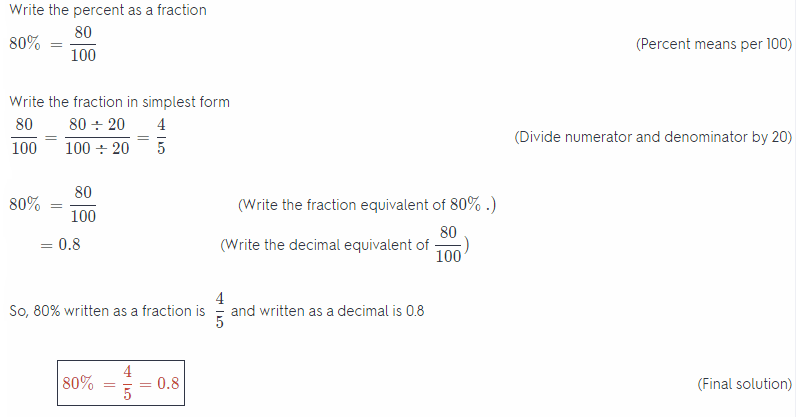80% = $$\frac{4}{5}$$ = 0.8

Question 4.
75% _______________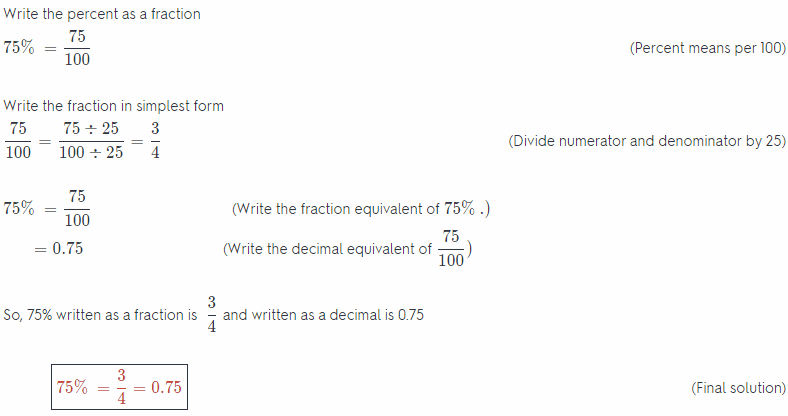75% = $$\frac{3}{4}$$ = 0.75

Question 5.
36% _______________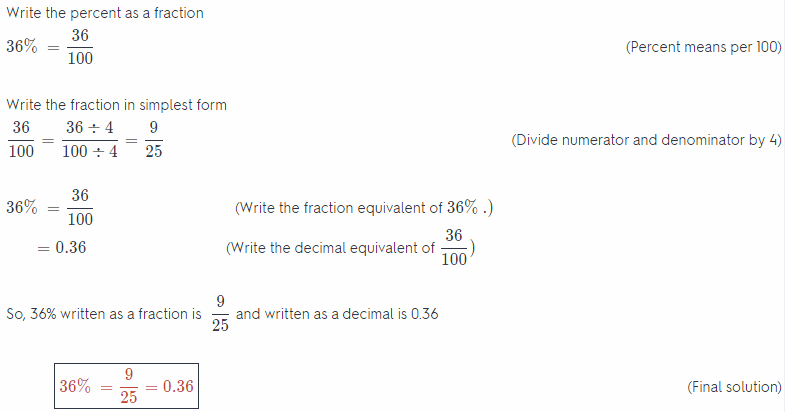36% = $$\frac{9}{25}$$ = 0.36

Question 6.
40% _______________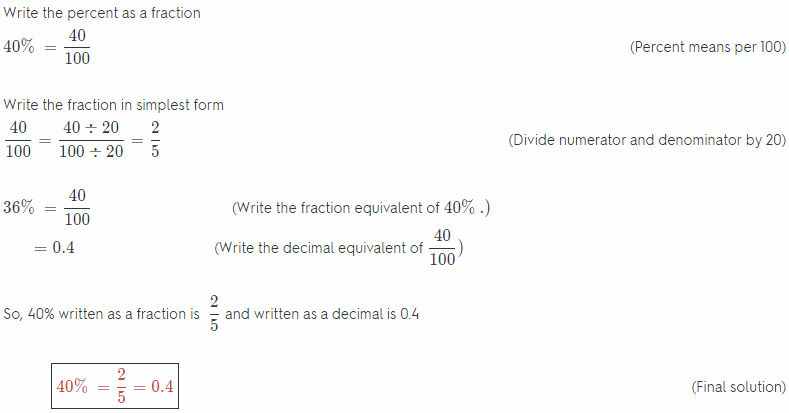40% = $$\frac{2}{5}$$ = 0.4

Reflect

Question 7.
Multiple Representations What decimal, fraction, and percent equivalencies are shown in each model? Explain.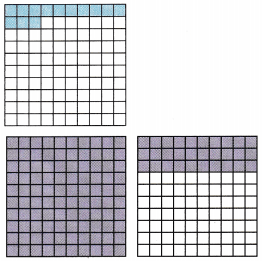a. _____________________________________
The first model is made of 100 boxes, 13 of which are shaded. So,
$$\frac{13}{100}$$ = 13 ÷ 100 = 0.13 = 13%
are the shown equivalencies

b. _____________________________________
The second model is made out of two 10 × 10 grids. The first is completely shaded, and the other one has 30 of 100 boxes shaded with the same color.
As in the example from the book right before this task, this model represents a percentage greater than 100%. So?
1 + $$\frac{30}{100}$$ = $$\frac{130}{100}$$ = 130% = 1.3 = $$\frac{13}{10}$$
are the shown equivalencies.

Write each fraction as a decimal and as a percent.

Question 8.
$$\frac{9}{25}$$ _______________
Write an equivalent fraction with a denominator of 100
$$\frac{9}{25}=\frac{9 \times 4}{25 \times 4}=\frac{36}{100}$$ (Multiply both the numerator and denominator by 4)

Write the decimal equivalent
$$\frac{36}{100}$$ = 0.36

Write the percent equivalent
$$\frac{36}{100}$$ = 0.36 = 0.36% (Move the decimal point 2 places to the right)

Final solution = $$\frac{9}{25}$$ = 0.36 = 0.36%

Question 9.
$$\frac{7}{8}$$ _______________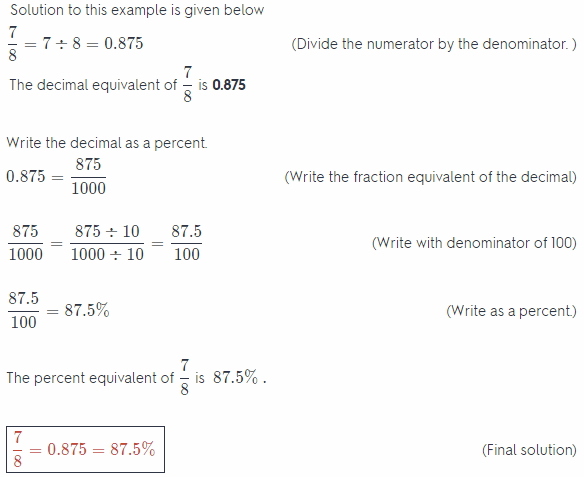Question 1.
Helene spends 12% of her budget on transportation expenses. Write this percent as a fraction and as a decimal.
Given percentage: = 12%

To convert it to an equivalent fraction replace the % sign with × $$\frac{1}{100}$$ and simplify:
= 12 × $$\frac{1}{100}$$
Divide out the common factor to simplify:
= $$\frac{3}{25}$$

Given percentage: = 36%
Convert the percentage to an equivalent decimal number by replacing the percentage sign with × $$\frac{1}{100}$$ The decimal equivalent of the given percentage or fraction is evaluated by moving the decimal 2 places to the left from its current position, therefore:
= 12 × $$\frac{1}{100}$$ = 0.12

Model the decimal. Then write percent and fraction equivalents.

Question 2.
0.530.53 = 53 ÷ 100 = $$\frac{53}{100}$$ = 53%
0.53 = $$\frac{53}{100}$$ = 53%

Question 3.
1.071.07 = 107 ÷ 100 = $$\frac{107}{100}$$ = 107%
1.07 = $$\frac{107}{100}$$ = 107%

Write each fraction as a decimal and as a percent.

Question 4.
$$\frac{7}{20}$$ of the packages __________________
Write an equivalent fraction with a denominator of 100
$$\frac{7}{20}=\frac{7 \times 5}{20 \times 5}=\frac{35}{100}$$ (Multiply both the numerator and denominator by 5)

Write the decimal equivalent
$$\frac{35}{100}$$ = 0.35

Write the percent equivalent.
$$\frac{35}{100}$$ = 0.35 = 35% (Move the decimal point 2 places to the right)
Final solution ⇒ $$\frac{7}{200}$$ = 0.35 = 0.35%

Question 5.
$$\frac{3}{8}$$ of a pie __________________
Divide the numerator by the denominator
$$\frac{3}{8}$$ = 3 ÷ 8 = 0.35
The decimal equivalent of $$\frac{3}{8}$$ is 0.375

Write the decimal as a percent.
0.3 75 = $$\frac{375}{1000}$$ (Write the fraction equivalent of the decimal)

$$\frac{375}{1000}=\frac{375 \div 10}{1000 \div 10}=\frac{37.5}{100}$$ (Write with denominator of 100)
$$\frac{37.5}{100}$$ = 37.5 % (Write as a percent)

The percent equivalent of $$\frac{3}{8}$$ is 37.5%.
Final solution ⇒ $$\frac{3}{8}$$ = 0.3 75 = 37.5%

Essential Question Check-In

Question 6.
How does the definition of percent help you write fraction and decimal equivalents?
The definition from page 203:
A percent is a ratio that compares a number to 100.
So, a ratio of a form x: 100 is called a percent and also written as x%
We also know how to convert ratios to fractions (the first term of the ratio is the numerator, and the second term is the denominator), and how to write fractions as decimal numbers (divide the numerator with the denominator)

So, we can conclude that
x% = x : 100 = $$\frac{x}{100}$$ = x ÷ 100
for any number x.

Write each percent as a fraction and as a decimal.

Question 7.
75% full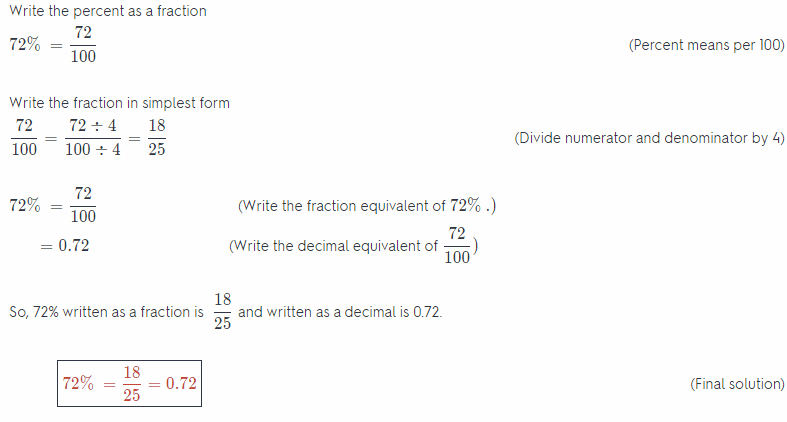Question 8.
25% successes
Given percentage: = 25%

Convert the percentage to an equivalent decimal number by replacing the percentage sign with × $$\frac{1}{100}$$. The decimal equivalent of the given percentage is evaluated by moving the decimal 2 places to the left from its current position, therefore:
= 25 × $$\frac{1}{100}$$ = 0.25

To convert it to an equivalent fraction replace the % sign with × $$\frac{1}{100}$$ and simplify:
= 25 × $$\frac{1}{100}$$
Divide out the common factor to simplify:
= $$\frac{1}{4}$$
25% = 0.25 =$$\frac{1}{4}$$

Question 9.
500% increase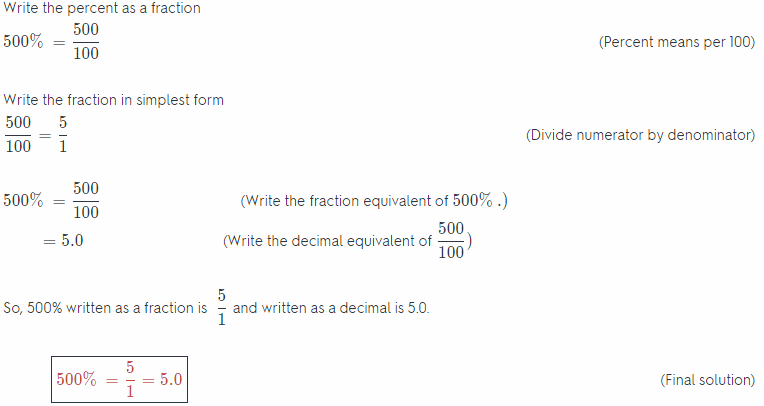Question 10.
5% tax
Given percentage: = 5%

Convert the percentage to an equivalent decimal number by replacing the percentage sign with × $$\frac{1}{100}$$ The decimal equivalent of the given percentage is evaluated by moving the decimal 2 places to the left from its current position, therefore:
= 5 × $$\frac{1}{100}$$ = 0.05

To convert it to an equivalent traction replace the % sign with × $$\frac{1}{100}$$ and simplify:
= 5 × $$\frac{1}{100}$$
Divide out the common factor to simplify:
= $$\frac{1}{20}$$
5% = 0.05 = $$\frac{1}{20}$$

Question 11.
37% profit.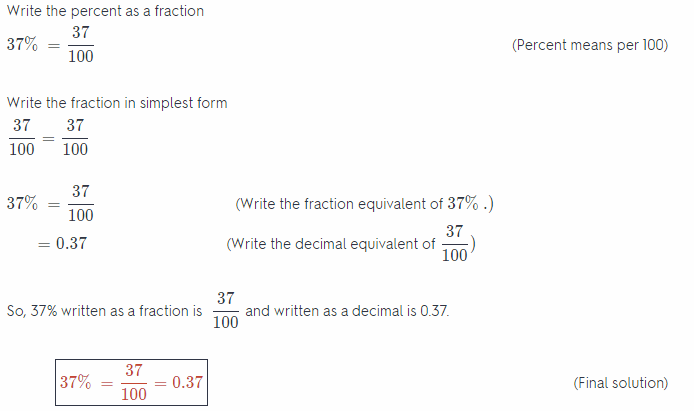Question 12.
165% improvement
Given percentage: = 165%

Convert the percentage to an equivalent decimal number by replacing the percentage sign with × $$\frac{1}{100}$$ The decimal equivalent of the given percentage is evaluated by moving the decimal 2 places to the left from its current position, therefore:
= 165 × $$\frac{1}{100}$$ = 1.65

To convert it to an equivalent traction replace the % sign with × $$\frac{1}{100}$$ and simplify:
= 165 × $$\frac{1}{100}$$
Divide out the common factor to simplify:
= $$\frac{33}{20}$$

165% = 1.65 = $$\frac{33}{20}$$

Write each fraction as a decimal and as a percent.

Question 13.
$$\frac{5}{8}$$ of an inch
Given fraction: = $$\frac{5}{8}$$

Multiply the given fraction with 100% to convert it to an equivalent percentage, therefore:
= $$\frac{5}{8}$$ × 100%

Evaluate:
62.5%

Convert the percentage to an equivalent decimal number by replacing the percentage sign with × $$\frac{1}{100}$$. The decimal equivalent of the given percentage or fraction is evaluated by moving the decimal 2 places to the left from its current position, therefore:
= 62.5 × $$\frac{1}{100}$$ = 0.625

⇒ $$\frac{5}{8}$$ = 62.5% = 0.625

Question 14.
$$\frac{258}{300}$$ of the contestants
Given fraction: = $$\frac{258}{300}$$

Multiply the given fraction with 100% to convert it to an equivalent percentage, therefore:
= $$\frac{258}{300}$$ × 100%

Evaluate:
86%

Convert the percentage to an equivalent decimal number by replacing the percentage sign with × $$\frac{1}{100}$$. The decimal equivalent of the given percentage or fraction is evaluated by moving the decimal 2 places to the left from its current position, therefore:
= 86 × $$\frac{1}{100}$$ = 0.86

⇒ $$\frac{258}{300}$$ = 86% = 0.86

Question 15.
$$\frac{350}{100}$$ of the revenue
Write the decimal equivalent
$$\frac{350}{100}$$ = 3.5 (Divide)

Write the percent equivalent
$$\frac{350}{100}$$ = 3.5 = 350% (Move the decimal point 2 places to the right)

Final solution ⇒ $$\frac{350}{100}$$ = 3.5 = 350%

Question 16.
The poster shows how many of its games the football team has won so far. Express this information as a fraction, a percent, and as a decimal.Total number of games played are 15 out of which 12 are won, so the fraction of wins is:
$$\frac{12}{15}$$

Multiply the given fraction with 100% to convert it to an equivalent percentage, therefore:
= $$\frac{12}{15}$$ × 100%

Evaluate:
= 80%

Convert the percentage to an equivalent decimal number by replacing the percentage sign with × $$\frac{1}{100}$$. The decimal equivalent of the given percentage or fraction is evaluated by moving the decimal 2 places to the left from its current position, therefore:
= 80 × $$\frac{1}{100}$$ = 0.8

⇒ $$\frac{12}{15}$$ = 80% = 0.8

Question 17.
Justine answered 68 questions correctly on an 80-question test. Express this amount as a fraction, percent, and decimal.
Total number of games played are 80 out of which 68 are answered, so the fraction of correct answer is:
$$\frac{68}{80}$$

Multiply the given fraction with 100% to convert it to an equivalent percentage, therefore:
= $$\frac{68}{80}$$ × 100%

Evaluate:
= 85%

Convert the percentage to an equivalent decimal number by replacing the percentage sign with × $$\frac{1}{100}$$. The decimal equivalent of the given percentage or fraction is evaluated by moving the decimal 2 places to the left from its current position, therefore:
= 85 × $$\frac{1}{100}$$ = 0.85

⇒ $$\frac{68}{80}$$ = 85% = 0.85

Each diagram is made of smaller, identical pieces. Tell how many pieces you would shade to model the given percent.

Question 18.
75% _________________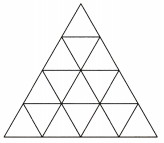Given percentage = 75%
There are a total of 16 small units in the figure, so in order to model the given percentage; $$\frac{75}{100}$$ × 16 = 12 of them will have to be shaded.

12 out of the 16 units will have to be shaded.

Question 19.
25% _________________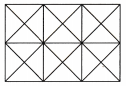Given percentage = 25%
There are a total of 16 small units in the figure, so in order to model the given percentage; $$\frac{25}{100}$$ × 24 = 6 of them will have to be shaded.

6 out of the 24 units will have to be shaded.

Question 20.
Multiple Representations At Brian’s Bookstore, 0.3 of the shelves hold mysteries, 25% of the shelves hold travel books, and $$\frac{7}{20}$$ of the shelves hold children’s books. Which type of book covers the most shelf space in the store? Explain how you arrived at your answer.
Convert all the given data to percentages so that they can be compared, therefore:

Given fraction: = $$\frac{7}{20}$$
Multiply the given fraction with 100% to convert it to an equivalent percentage, therefore:
= $$\frac{7}{20}$$ × 100%

Evaluate:
= 35%

Convert the decimal number to an equivalent percentage by multiplying it with 100%. Note that the decimal will move 2 places to the right from its current position, therefore:
= 0.3 × 100% = 30%
Here 35% > 30% > 25%, therefore it can be said that children’s books hold the most shelf space.

Children’s books hold the most shelf space.

H.O.T. Focus On Higher Order Thinking

Question 21.
Critical Thinking A newspaper article reports the results of an election between two candidates. The article says that Smith received 60% of the votes and that Murphy received $$\frac{1}{3}$$ of the votes. A reader writes in to complain that the article cannot be accurate. What reason might the reader have to say this?
The fraction $$\frac{1}{3}$$ = $$\frac{1}{3}$$ × 100% = 33.$$\overline{3}$$% The 2 resuLts of the elections should add up to 100%, here this is not the case, so the article must be incorrect.

The results do not add up to 100% or 1.

Question 22.
Represent Real-World Problems Evan budgets $2,000 a month to spend on living expenses for his family. Complete the table to express the portion spent on each cost as a percent, fraction, and decimal.Answer:  Food:$500 Rent: $1200 Transportation:$300 Fraction $$\frac{500}{2000}=\frac{1}{4}$$ $$\frac{1200}{2000}=\frac{3}{5}$$ $$\frac{300}{2000}=\frac{3}{20}$$ Percent $$\frac{1}{4}$$ × 100% = 25% $$\frac{3}{5}$$ × 100% = 60% $$\frac{3}{20}$$ × 100% = 15% Decimal $$\frac{25}{100}$$ = 0.25 $$\frac{60}{100}$$ = 0.6 $$\frac{15}{100}$$ = 0.15

Question 23.
Communicate Mathematical Ideas Find the sum of each row in the table. Explain why these sums make sense.
The sum of the 1st row is $$\frac{1}{4}+\frac{3}{5}+\frac{3}{20}$$ = 1 The sum of fractions add up to 1. It can be said that the total budget is 1 and each fraction is a portion of that total budget.

The sum of the 2nd row is 25% + 60% + 15% = 100%. The sum of percents add up to 100%. It can be said that the total budget is 100% and each percent is a portion of that total budget.

The sum of the 3rd row is 0.25 + 0.6 + 0.15 = 1. The sum of decimal numbers add up to 1. It can be said that the total budget is 1 and each decimal numbers is a portion of that total budget.

Question 24.
Explain the Error Your friend says that 14.5% ¡s equivalent to the decimal 14.5. Explain why your friend is incorrect by comparing the fractional equivalents of 14.5% and 14.5.
Convert the percentage to an equivalent decimal number by repLacing the percentage sign with × $$\frac{1}{100}$$ The decimal equivalent of the given percentage is evaluated by moving the decimal 2 places to the left from its current position, therefore:
= 14.5 × $$\frac{1}{100}$$ = 0.145 ≠ 14.5